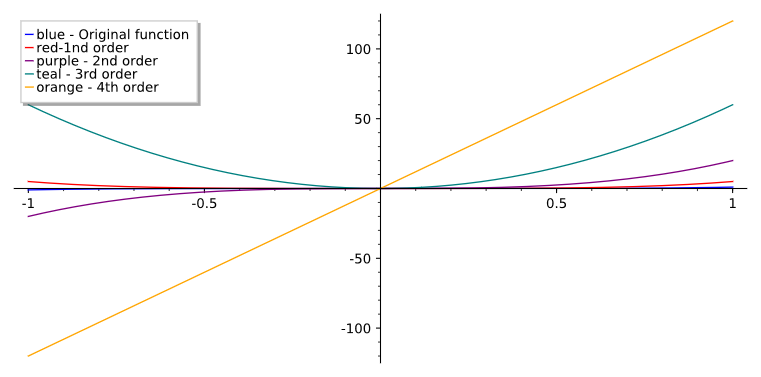Author Aaron E-J Date 2019-08-16T17:27:13 Project 7bf42edc-de86-436d-a9e4-0fece1d91ec8 Original file Differentiation.sagews
%autotypeset_mode(True)var('x y z k w')
($\displaystyle x$, $\displaystyle y$, $\displaystyle z$, $\displaystyle k$, $\displaystyle w$)

Derivative – A variable that describes the amount of change at a given function's output value with respect to its input value.

Differential = infinitesimal change in some varying quantity

Differentiation = a function describing the rate of change of another function; the method by which a derivative is found for a given funtion

Differential Equation = an equation that describes the relationship between a function and it's derivative

Function:

#a sample function, x to the power of 5f(x)=x^5;f
$\displaystyle x \ {\mapsto}\ x^{5}$

Derivative:

#1st orderderivative(f,x)#Shortened spelling that is not auto run but stored as a variable:D=diff(f,x)#2nd orderdiff(f,x,x)#3rd orderdiff(f,x,x,x)#4th orderdiff(f,x,x,x,x)#5th order, Notice the numerical format, this could be replaced by adding 4 more 'x'sdiff(f,x,5)
$\displaystyle x \ {\mapsto}\ 5 \, x^{4}$
$\displaystyle x \ {\mapsto}\ 20 \, x^{3}$
$\displaystyle x \ {\mapsto}\ 60 \, x^{2}$
$\displaystyle x \ {\mapsto}\ 120 \, x$
$\displaystyle x \ {\mapsto}\ 120$

Graphing the previous function and its derivative:

#add line to graph the functionp=plot(f,x, legend_label='blue - Original function')#add lines to graph the derivativesp+=plot(D,x,color="red",legend_label='red-1nd order')p+=plot(derivative(f,x,x),x,color="purple",legend_label='purple - 2nd order')p+=plot(derivative(f,x,x,x),x,color="teal",legend_label='teal - 3rd order')p+=plot(derivative(f,x,4),x,color="orange",legend_label='orange - 4th order')p